# k-Nearest Neighbors

The advantages of the k-NN approach are that:

• It is simple and easy to understand why a particular prediction is made.
• A k-NN approach can be a reasonable baseline against which you can compare more sophisticated methods.

The disadvantages of the k-NN model are that:

• When the training dat has many instances, or each instance has lots of features, the k-NN model may slow down significantly. If your dataset has hundreds or thousands of features, especially if they are sparse, consider alternatives.

The key parameters in both classification and regression models are:

• n_neighbors: the number of nearest neighbors (k) to consider, which directly controls model complexity.
• Default = 5
• metric: the function that computes the distance between data points, which controls which neighbors are calculated as nearest.
• Default = Minkowski distance with power parameter 2 (Euclidean), which works well for most datasets.

### The k-Nearest Neighbor (k-NN) Classifier Algorithm

Given a training set $X_{train}$ with labels $y_{train}$, and given a new instance $X_{test}$ to be classified:

1. Find the most similar instances (called $X_{NN}$) to $X_{test}$ that are in $X_{train}$.

2. Get the labels $y_{NN}$ for the instances in $X_{NN}$.

3. Predict the label for $X_{test}$ by combining the labels $y_{NN}$ by, for example, simple majority vote.

### Low k leads to Overfitting

As shown in the image below, a too-low value for k will result in jagged, high variance decision boundaries. This is because for k = 1, the regions that are nearest to each training point will have the same class as that training point.

This is an example of a classification model that has high model complexity.

plot_two_class_knn(X_train, y_train, 1, 'uniform', X_test, y_test)

<IPython.core.display.Javascript object>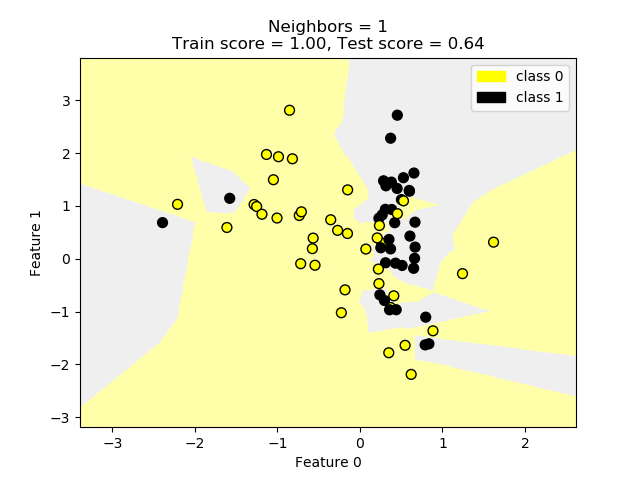Contrast this with k = 3, and k = 11, which have much smoother boundaries. Because the value of k is higher, the estimator is required to take into account many more points. The net result is that a single training data point has much less influence on the prediction, overall.

The result is a smoother decision boundary that better captures the overall global trends. The model’s generalization ability has improved.

These overall trends, from overfit to better fit to excellent fit, are born out in some of the accuracy measures in the titles of these plots. These are tabulated below. Note that the testing accuracy increases with increasing k, ultimately exceeding the training accuracy. This indicates successful generalization to the broader dataset.

k Training Accuracy Testing Accuracy
1 100% 64%
3 80% 72%
11 73% 80%
plot_two_class_knn(X_train, y_train, 3, 'uniform', X_test, y_test)
plot_two_class_knn(X_train, y_train, 11, 'uniform', X_test, y_test)

<IPython.core.display.Javascript object>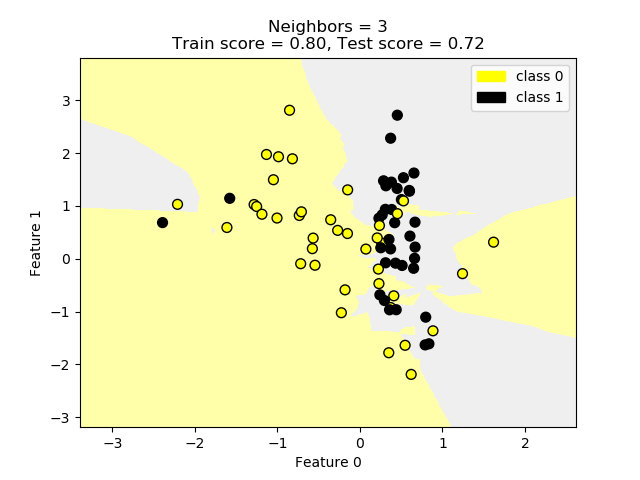<IPython.core.display.Javascript object>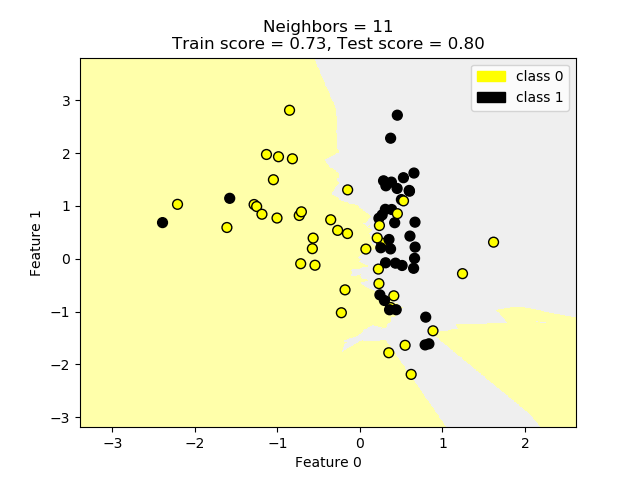### k-NN for Regression

• The $R^2$ (“r-squared”) Regression Score measures how well a prediction model for regression fits the given data. This is also known as the “coefficient of determination.”

• The score is between 0 and 1:

• A value of 0 corresponds to a constant model that predicts the mean value of all training target values.
• A value of 1 corresponds to perfect prediction.
print('R-squared test score: {:.3f}'
.format(knnreg.score(X_test, y_test)))

R-squared test score: 0.425

plot_regression_curves_given_k()

<IPython.core.display.Javascript object>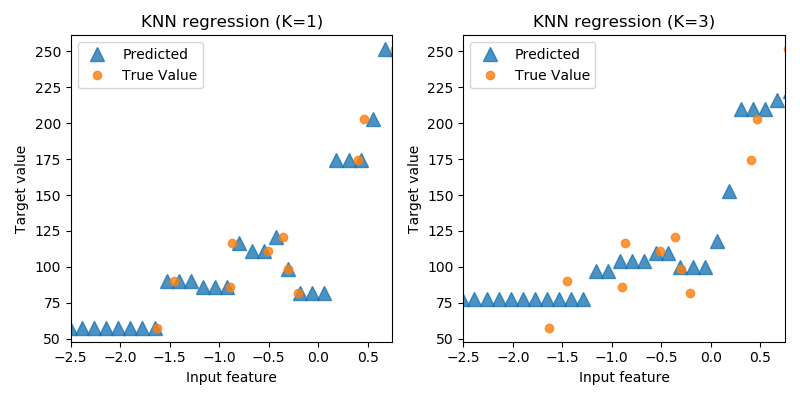Again, note that for increasing values of k, the value of testing $R^2$ increases, reaching a maximum with k = 15.

k Training $R^2$ Testing $R^2$
1 1.000 0.155
3 0.797 0.323
7 0.720 0.471
15 0.647 0.485
55 0.357 0.371

For the extreme value of k = 55, performance decreases, indicating an underfit.

plot_regression_model_complexity()

<IPython.core.display.Javascript object>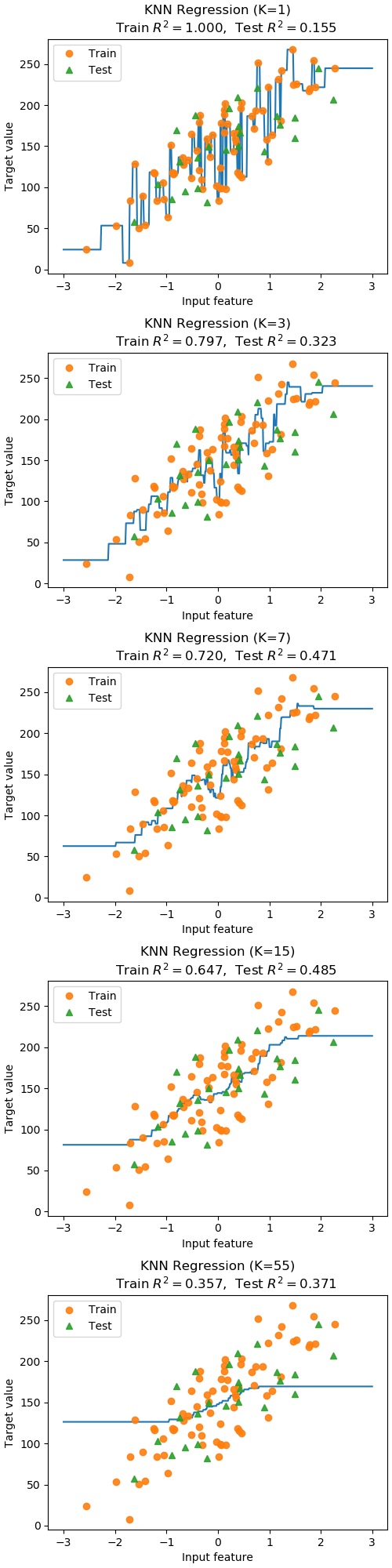### Utility Functions for Plots Above

#### Library Imports

%matplotlib notebook

import numpy as np
import matplotlib
import pandas as pd
import sklearn

print('       Numpy version ' + str(np.__version__))
print('  Matplotlib version ' + str(matplotlib.__version__))
print('      Pandas version ' + str(pd.__version__))
print('     Sklearn version ' + str(sklearn.__version__))

       Numpy version 1.18.1
Matplotlib version 3.1.1
Pandas version 0.25.3
Sklearn version 0.22.1

import matplotlib.pyplot as plt

from matplotlib.colors import ListedColormap
import matplotlib.patches as mpatches

from sklearn.datasets import make_classification
from sklearn.model_selection import train_test_split
from sklearn.neighbors import KNeighborsClassifier

from sklearn.datasets import make_regression
from sklearn.neighbors import KNeighborsRegressor


#### Low k leads to Overfitting

X_C2, y_C2 = make_classification(n_samples = 100,
n_features=2,
n_redundant=0,
n_informative=2,
n_clusters_per_class=1,
flip_y = 0.20,
class_sep = 0.5,
random_state=0)

X_train, X_test, y_train, y_test = train_test_split(X_C2, y_C2, random_state=0)

def plot_two_class_knn(X, y, n_neighbors, weights, X_test, y_test):
X_mat = X
y_mat = y

# Create color maps
cmap_light = ListedColormap(['#FFFFAA', '#AAFFAA', '#AAAAFF','#EFEFEF'])
cmap_bold  = ListedColormap(['#FFFF00', '#00FF00', '#0000FF','#000000'])

clf = KNeighborsClassifier(n_neighbors,
weights=weights)

clf.fit(X_mat, y_mat)

# Plot the decision boundary by assigning a color in the color map
# to each mesh point.

mesh_step_size = .01  # step size in the mesh
plot_symbol_size = 50

x_min, x_max = X_mat[:, 0].min() - 1, X_mat[:, 0].max() + 1
y_min, y_max = X_mat[:, 1].min() - 1, X_mat[:, 1].max() + 1
xx, yy = np.meshgrid(np.arange(x_min, x_max, mesh_step_size),
np.arange(y_min, y_max, mesh_step_size))
Z = clf.predict(np.c_[xx.ravel(), yy.ravel()])

# Put the result into a color plot
Z = Z.reshape(xx.shape)
plt.figure()
plt.pcolormesh(xx, yy, Z, cmap=cmap_light)

# Plot training points
plt.scatter(X_mat[:, 0],
X_mat[:, 1],
s=plot_symbol_size,
c=y,
cmap=cmap_bold,
edgecolor = 'black')
plt.xlim(xx.min(), xx.max())
plt.ylim(yy.min(), yy.max())

title = "Neighbors = {}".format(n_neighbors)
if (X_test is not None):
train_score = clf.score(X_mat, y_mat)
test_score  = clf.score(X_test, y_test)
title = title + "\nTrain score = {:.2f}, Test score = {:.2f}".format(train_score, test_score)

patch0 = mpatches.Patch(color='#FFFF00', label='class 0')
patch1 = mpatches.Patch(color='#000000', label='class 1')
plt.legend(handles=[patch0, patch1])

plt.xlabel('Feature 0')
plt.ylabel('Feature 1')
plt.title(title)

plt.show()


#### k-NN for Regression

X_R1, y_R1 = make_regression(n_samples = 100, n_features=1,
n_informative=1, bias = 150.0,
noise = 30, random_state=0)

X_train, X_test, y_train, y_test = train_test_split(X_R1, y_R1, random_state = 0)

knnreg = KNeighborsRegressor(n_neighbors = 5).fit(X_train, y_train)

def plot_regression_curves_given_k():
fig, subaxes = plt.subplots(1, 2, figsize=(8,4))
X_predict_input = np.linspace(-3, 3, 50).reshape(-1,1)
X_train, X_test, y_train, y_test = train_test_split(X_R1[0::5], y_R1[0::5], random_state = 0)

for thisaxis, K in zip(subaxes, [1, 3]):
knnreg = KNeighborsRegressor(n_neighbors = K).fit(X_train, y_train)
y_predict_output = knnreg.predict(X_predict_input)
thisaxis.set_xlim([-2.5, 0.75])
thisaxis.plot(X_predict_input, y_predict_output, '^', markersize = 10,
label='Predicted', alpha=0.8)
thisaxis.plot(X_train, y_train, 'o', label='True Value', alpha=0.8)
thisaxis.set_xlabel('Input feature')
thisaxis.set_ylabel('Target value')
thisaxis.set_title('KNN regression (K={})'.format(K))
thisaxis.legend()
plt.tight_layout()

def plot_regression_model_complexity():
fig, subaxes = plt.subplots(5, 1, figsize=(5,20))
X_predict_input = np.linspace(-3, 3, 500).reshape(-1,1)
X_train, X_test, y_train, y_test = train_test_split(X_R1, y_R1,
random_state = 0)

for thisaxis, K in zip(subaxes, [1, 3, 7, 15, 55]):
knnreg = KNeighborsRegressor(n_neighbors = K).fit(X_train, y_train)
y_predict_output = knnreg.predict(X_predict_input)
train_score = knnreg.score(X_train, y_train)
test_score = knnreg.score(X_test, y_test)
thisaxis.plot(X_predict_input, y_predict_output)
thisaxis.plot(X_train, y_train, 'o', alpha=0.9, label='Train')
thisaxis.plot(X_test, y_test, '^', alpha=0.9, label='Test')
thisaxis.set_xlabel('Input feature')
thisaxis.set_ylabel('Target value')
thisaxis.set_title('KNN Regression (K={})\n\
Train $R^2 = {:.3f}$,  Test $R^2 = {:.3f}$'
.format(K, train_score, test_score))
thisaxis.legend()